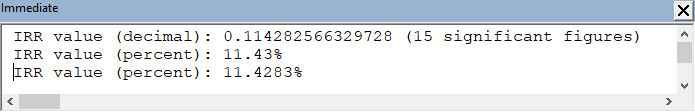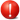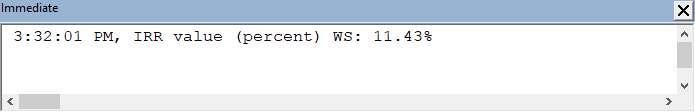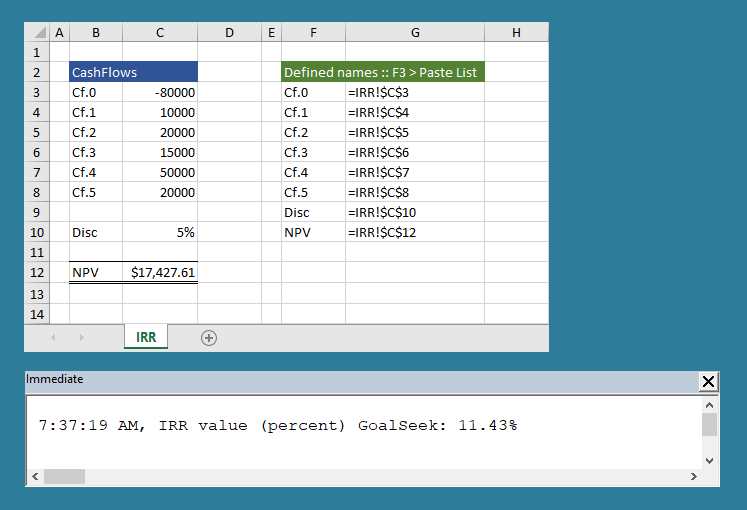# VBA financial :: IRR

## 0. Introduction to cash flow functions

All examples are based on the xlf Carrot Washer project link.

## 1. Internal rate of return (IRR)

The internal rate of return (IRR) for a series of cash flows, is the discount rate that equates to a zero net present value (NPV).

In equation form the NPV of 0 is set equal to the sum of discounted cash flows $$\begin{equation} 0=\sum_{t=0}^{n} \frac{C_t}{(1+IRR)^t} \end{equation}$$ where the cash flows at time $t$ are denoted $C_t$ over $n$ periods. Equation 1 is solved for $IRR$.

### 1.1 IRR VBA function

SYNTAX (VBA): IRR(ValueArray() As Double, [Guess]) As Double

Code 1: Sub DemoIRR returns the NPV for the carrot washer project using the VBA IRR function (VBA.IRR)
Option Explicit

' =================
' IRR VBA function
' =================
Sub DemoIRR()
' procedure declarations
Dim CashFlows(0 To 5) As Double ' << Type [Cashflows array] must be Double
Dim IrrDec As Double

' assignment statements
CashFlows(0) = -80000
CashFlows(1) = 10000
CashFlows(2) = 20000
CashFlows(3) = 15000
CashFlows(4) = 50000
CashFlows(5) = 20000

' calculation
IrrDec = IRR(CashFlows)
Debug.Print Time & ", IRR value (decimal): " & IrrDec & " (15 significant figures)"
Debug.Print Time & ", IRR value (percent): " & Format(IrrDec, "percent")
Debug.Print Time & ", IRR value (percent): " & Format(IrrDec, "0.0000%")

End SubFig 1: Immediate window - calculate the internal rate of return for the carrot washer project with the VBA IRR function (time stamp suppressed)Type [Cashflows array] - the array Type, code 1 line 8 must be a Double
Other data types such as Variant or Single return a Compile error: Type mismatch array or user-defined type expected at line 20

### 1.2 IRR WS function

SYNTAX (WS): IRR(values, [guess])

Pass cash flow values from a VBA array.

Code 2: Sub DemoIRRws returns the NPV for the carrot washer project using the (WS) IRR function (Application.IRR)
' =================
' IRR WS function
' =================
Sub DemoIRRws()
' procedure declarations
Dim CashFlows(0 To 5) As Double
Dim IrrDec As Double

' assignment statements
CashFlows(0) = -80000
CashFlows(1) = 10000
CashFlows(2) = 20000
CashFlows(3) = 15000
CashFlows(4) = 50000
CashFlows(5) = 20000

' calculation
IrrDec = Application.IRR(CashFlows)
Debug.Print Time & ", IRR value (percent) WS: " & Format(IrrDec, "percent")

End SubFig 2: Immediate window - calculate the internal rate of return for the carrot washer project with the WS IRR function

### 1.3 IRR GoalSeek method

SYNTAX (VBA): Range().GoalSeek Goal:=value, ChangingCell:=Range(). Returns TRUE or FALSE

Cash flow values are stored in a worksheet range.

Code 3: Sub DemoIRRgs returns the NPV for the carrot washer project using the VBA Range.GoalSeek method
 =================
' IRR GoalSeek method
' =================
Sub DemoIRRgs()

Dim IrrDec As Double

' reset disc rate
Range("Disc").Value = "5%"

' calculation
Range("NPV").GoalSeek Goal:=0, ChangingCell:=Range("Disc")
IrrDec = Range("Disc")
Debug.Print vbNewLine & Time & ", IRR value (percent) GoalSeek: " & Format(IrrDec, "percent")

End SubFig 3: Immediate window - calculate the internal rate of return for the carrot washer project with the Range.GoalSeek method